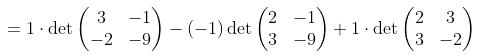December 1, 2020

# Introduction To Matrices And Solving Linear Equations Using Matrices

A matrix is the arrangement of numbers in rows and columns. The representation of a...

A matrix is the arrangement of numbers in rows and columns. The representation of a matrix is as follows:

An m × n matrix consists of m rows which are horizontal and the n columns which are vertical.

Basic operations of a matrix involve matrix addition, scalar multiplication, transpose, matrix multiplication and row operations. The order of a matrix is defined as the number of rows and columns that are present in the matrix. The different types of matrices are square, diagonal, symmetric, skew-symmetric, unit, orthogonal, idempotent matrices. The transpose of a matrix is obtained when the rows and columns of the matrix are interchanged.

The equations of the first order can be termed as linear equations. The highest exponent of these first-degree equations is 1. There are different methods to solve the system of equations namely graphing method,  substitution method, elimination method, Cramer’s rule, Gaussian elimination. One of the methods is solving linear equations using matrices.

The linear equations can be represented in the form of a matrix as follows.

Consider the set of equations

a1x + b1y + c1z = d1

a2x + b2y + c2z = d2

a3x + b3y + c3z = d3

Steps to find the solution for the system of linear equations

Step 1: The given equations should be written in the standard form ax + by + c = d.

Step 2: The variables, coefficients and the constants should be written in an appropriate order.

Step 3: The given set of equations is written in the form of AX = B, where A represents the coefficient matrix, X represents the matrix of variables and B represents the constant matrix.

Step 4: The determinant of the matrix A is calculated.

Step 5: The adjoint matrix is the transpose of the cofactor matrix. The matrix of cofactors is obtained by calculating the determinant by eliminating the row and column of a particular element of a matrix.

Step 6: The inverse matrix is found using the formula A-1 = adjoint matrix [adj A] / determinant matrix [|A|] or by row elimination method.

Step 7: The value of the unknowns can be calculated using the formula X = A-1B.

Example 1: Solve the system of equations by matrix inversion method.

x − y + z = 8

2x + 3y − z = −2

3x − 2y − 9z = 9

Solution:

The given equations are in the standard form.

They can be represented in the form of AX = B.

|A| == 1 [3 * (-9) – (-1 * -2)] – [(-1) (2 * -9 – (-1 * 3))] + [1 (2 * -2 – 3 * 3)]

= 1 (-27 – 2) – (-1) (-18 + 3) + 1 (-4 – 9)

= -29 – 15 – 13

|A| = -57

To find the inverse of matrix A, we need to find the matrix of cofactors.

The values of the unknowns are x = 4, y = -3, z = 1.

Types of Solutions of a System of Linear Equations

Consistent and Inconsistent System Of Equations

If the system of equations consists of one or more solutions, then it is said to be a consistent system of equations, else, it is an inconsistent system of equations.

The three possible cases of solutions are:

• No solution
• One solution
• Infinitely many solutions

Condition for consistency of a system of linear equation AX = B

(a) If |A| ≠ 0, then the system is consistent and has a unique solution, given by X = A−1B

(b) If |A| = 0, and (Adj A) B ≠ 0 then the system is inconsistent.

(c) If |A| = 0, and (Adj A) B = 0, then the system is consistent and has infinitely many solutions.

#### You may have missed#### Three Causes Software Testing Delivers Better Quality For Much less Cash